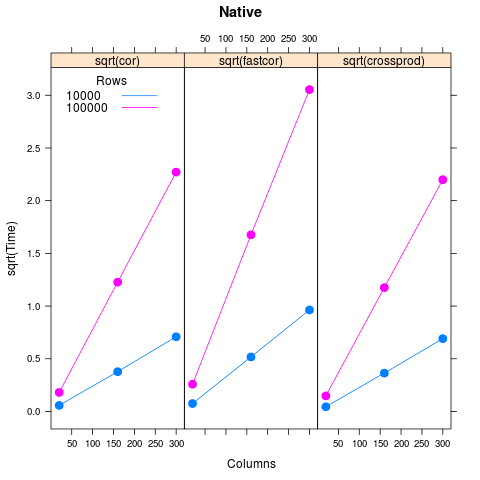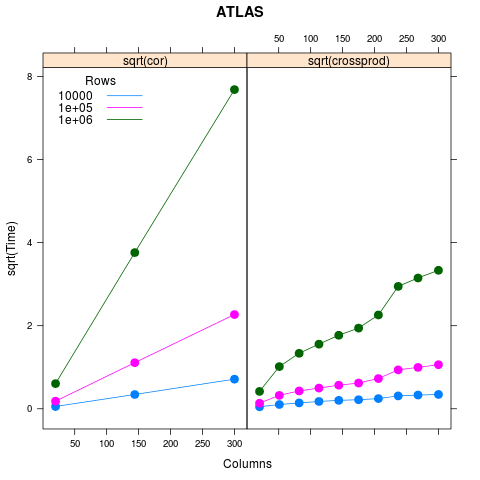# Parallel evaluation and memory managment for high performance computing

## Parallel evaluation

### Approaches

• Parallel linear algebra libraries
• See case study, below
• (non-Windows) forked processes
• Socket and other ad hoc clusters
• High-performance MPI clusters

### Interfaces

ATLAS, ACML BLAS library

• Great for efficient and parallel linear algebra routines
• Transparent use
• Building R with user BLAS; tricky but not impossible.

'parallel' package

• multi-core
• mclapply and friends: parallel computation on lists (lapply(...))
• pvec: parallel computations on vectors (fun(...))
• mcparallel, mccollect: fork and retrieve
• Shared memory, copy-on-write
• Simple Network of Workstations (SNOW)
• Communication via sockets
• Manager-worker model: single R process spawns workers
• Distinct processes, so each worker needs to be made the same
• Easy to use; cross-platform

Rmpi

• MPI interface
• Manager / worker model
• Spawn, lapply-like, etc
• Easy to use interactively
• Pool of available hosts can be managed by mpi (via e.g., mpirun, a StackOverflow example), which makes sense (responsibility for managing cluster structure should not be R's responsibility).
• Also 'single instruction / multiple data' style
• Better than manager worker: MPI and cluster management software manage jobs
• Example: pbdR

foreach

• Iteration (yuck!) with plug-in back-ends (yum!)

BatchJobs

• Managing queues

BiocParallel

• Common interface, e.g., bplapply for any back-end
• Registration of current back end, so automatic selection
• More sensible defaults and implementations

### Case study: parallel linear algebra

Motivation: StackOverflow question about calculating correlation coefficients between columns in a large (1M x 400) numeric matrix.

• Plain R: How does cor() scale? Some set-up:

set.seed(123)
timer <- function(dim, FUN, nrep=3) {
print(dim)
m <- matrix(runif(dim * dim), dim)
mean(replicate(nrep, system.time(FUN(m))["elapsed"]))
}
parm <- expand.grid(m=10^(4:5),
n=as.integer(seq(20, 300, length.out=3)))


And then calculation:

parm$cor <- apply(parm[,1:2], 1, timer, cor) xtabs(cor ~ m + n, parm)  • A little math: (1) center; (2) standardize; (3) cross-product. Step (3) is the slow part, and is performed by R's math library. Implementation: fastcor <- function(m) { m <- t(m) m <- m - rowMeans(m) # center m <- m / sqrt(rowSums(m^2)) # scale tcrossprod(m) # cross-product }  How are we doing? ## 'small' data set initially m <- 100000; n <- 50 mat <- matrix(runif(m * n), m)  Timing and correctness: system.time(c0 <- cor(mat))  ## user system elapsed ## 0.49 0.00 0.49  system.time(c1 <- fastcor(mat))  ## user system elapsed ## 0.270 0.025 0.314  all.equal(c0, c1) # why not identical()?  ##  TRUE  Scaling: parm$fastcor <- apply(parm[,1:2], 1, timer, fastcor)
parm$crossprod <- apply(parm[,1:2], 1, timer, crossprod)  Visualizing: library(lattice) xyplot(sqrt(cor) + sqrt(fastcor) + sqrt(crossprod) ~ n, group=m, parm, type="b", pch=20, cex=2, layout=c(3, 1), xlab="Columns", ylab="sqrt(Time)", main="Native", key=simpleKey(text=sprintf("%d", unique(parm$m)), lines=TRUE,
points=FALSE, x=.02, y=.95, title="Rows", cex.title=1))Same scaling, worse coefficient, worse algorithm!

• But wait! Use a parallel BLAS library:• Initial diminishing gains – multiple processor
• Linear end – implementation (configurable?); better coefficient

'Easy' to arrange for parallel evaluation on Linux / Mac: (1) install appropriate library using a package manager, e.g., apt get install libatlas3gf-base; (2) download R source and configure to use installed library (see R Installation and Administration); (3) make -j; (4) Use.

## Memory management

Basic observations

• Embarassingly parallel problems require parallel solution because of volume of data, rather than complexity of algorithm
• On a single machine, using more cores implies each cores has access to less memory
• Expensive to move data from manager to worker
• Conclusion: memory management is an integral part of high performance computing

Approach

• Use file system as a passive way to make data available across a cluster
• Organize data in a way that allows slices to be drawn in to memory
• data base (especially relational data resources)
• rhdf5, especially for array data common in scientific programming
• R-specific solutions, e.g., ff, bigmemory. Used by, e.g., oligo
• Domain-specific solutions, e.g., indexed BAM files

Common solutions 1. Restrict data input to just that required 2. Draw a sample and infer statistical properties if appropriate, e.g., QA 3. Iterate through large data

• In chunks of many 100k's rows, to use R's efficient vector operations
• Examples of use: Rsamtools::filterBam, GenomicRanges::summarizeOverlaps
1. Combine efficient code + 1-3 with parallel evaluation

Case study: Counting reads overlapping regions of interest, Intermediate Sequence Analysis 2013 Chapter 7.